# 11 3 Prisms Cylinders FGuilbert mathbyart com Volume

• Slides: 22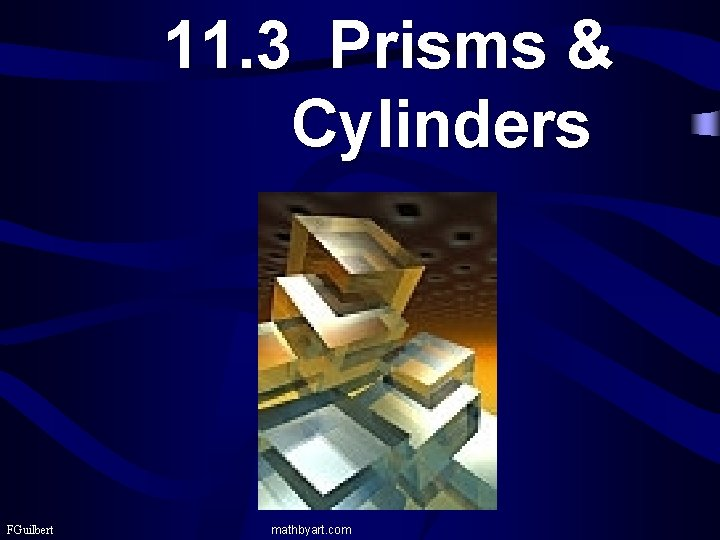11. 3 Prisms & Cylinders FGuilbert mathbyart. com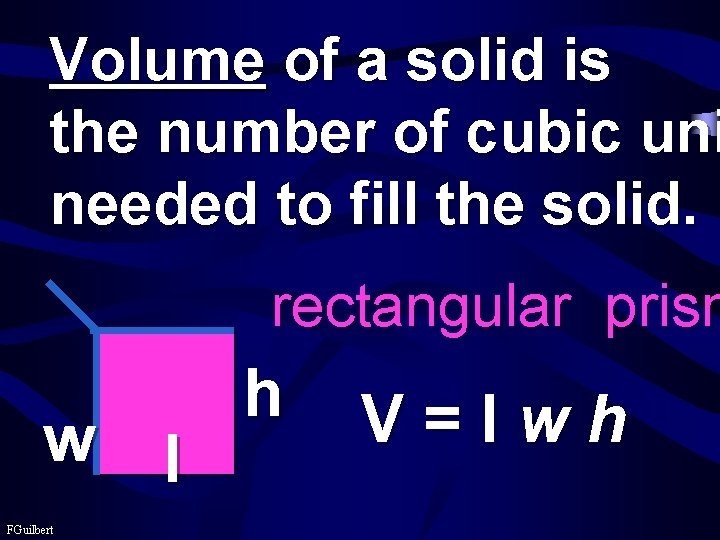Volume of a solid is the number of cubic uni needed to fill the solid. rectangular prism w FGuilbert l h V=lwh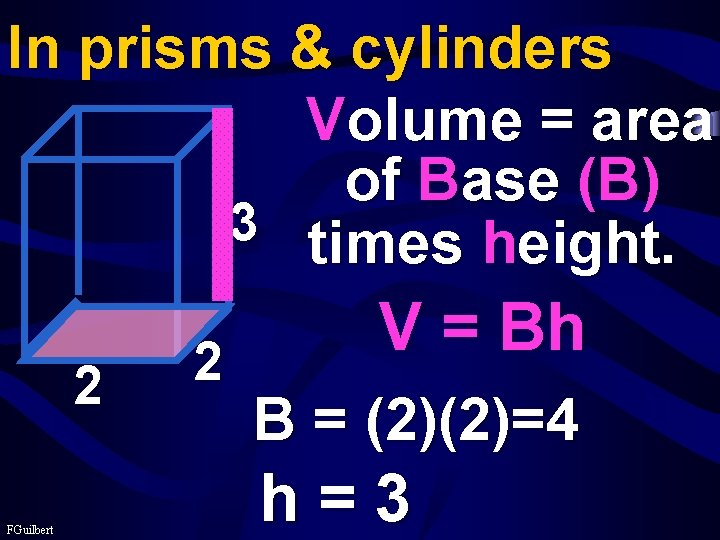In prisms & cylinders Volume = area of Base (B) 3 times height. 2 FGuilbert 2 V = Bh B = (2)(2)=4 h=3In prisms & cylinders Volume = area of Base (B) 3 times height. 2 2 V = Bh # cubic units in a figure FGuilbertMaybe you need to know many cranberries fit in a b FGuilbertV = Bh area base B=4 3 2 FGuilbert 2 h=3 V= (4)(3) = 12 12 cubic units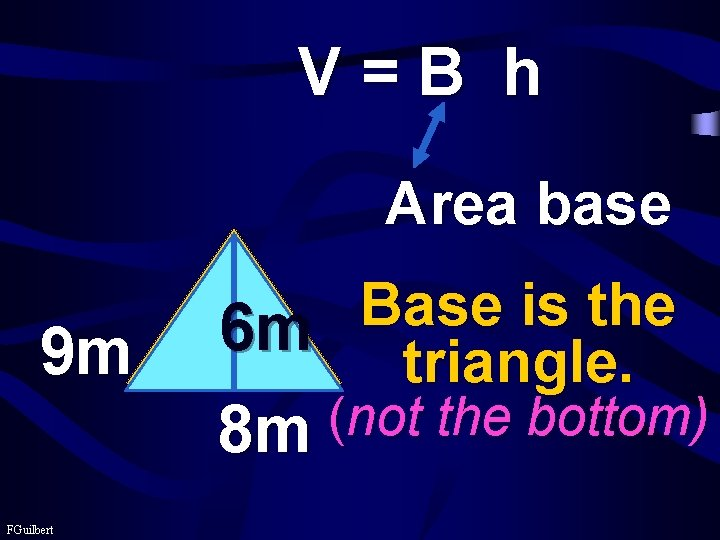V=B h Area base 9 m FGuilbert Base is the 6 m triangle. ( not the bottom) 8 m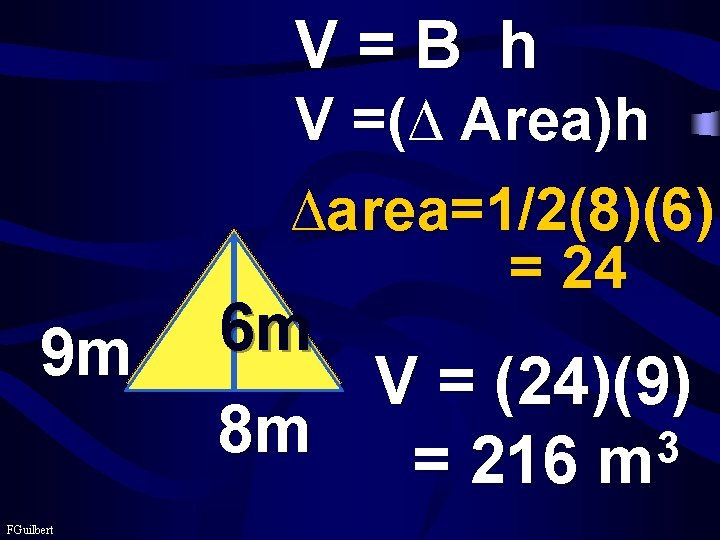V=B h V =(∆ Area)h ∆area=1/2(8)(6) = 24 9 m FGuilbert 6 m V = (24)(9) 8 m 3 = 216 m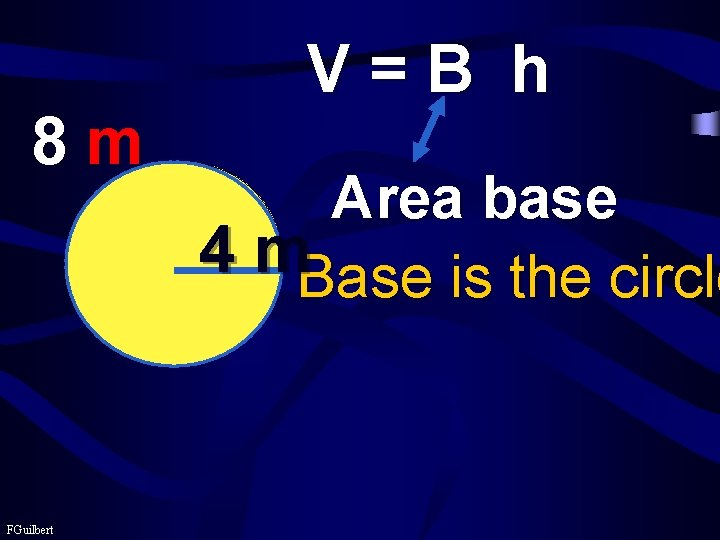8 m FGuilbert V=B h Area base 4 m. Base is the circleV=B h 8 FGuilbert 4 m 2 4 8 V= π 3 V= 128 π m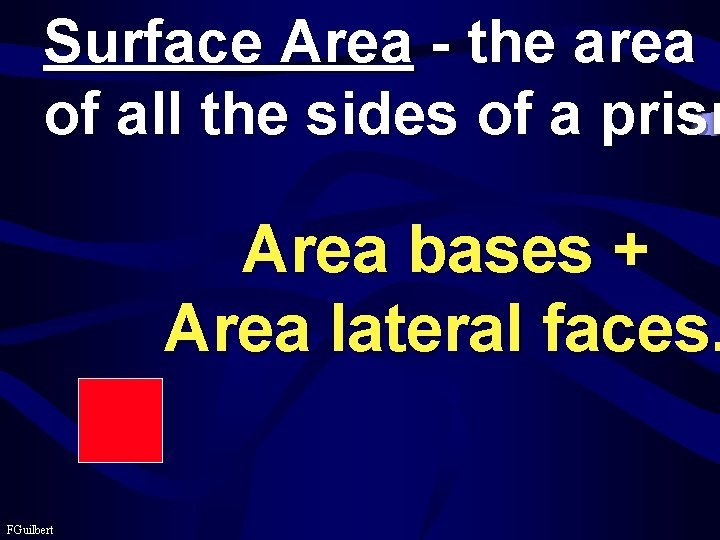Surface Area - the area of all the sides of a prism Area bases + Area lateral faces. FGuilbert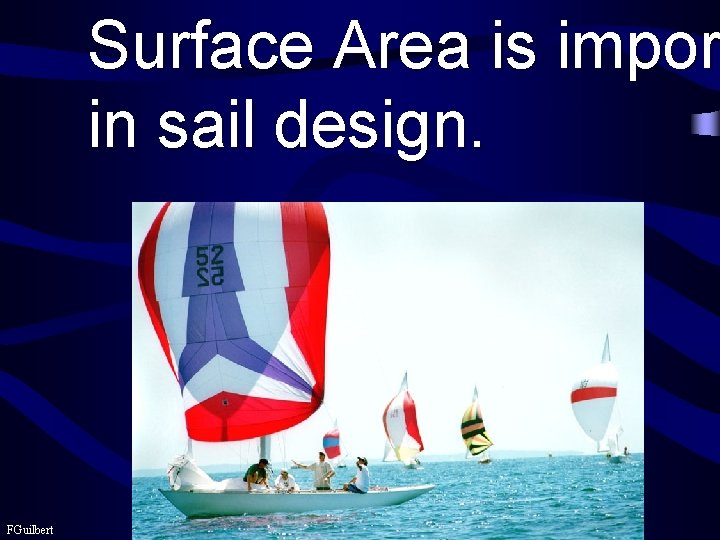Surface Area is impor in sail design. FGuilbert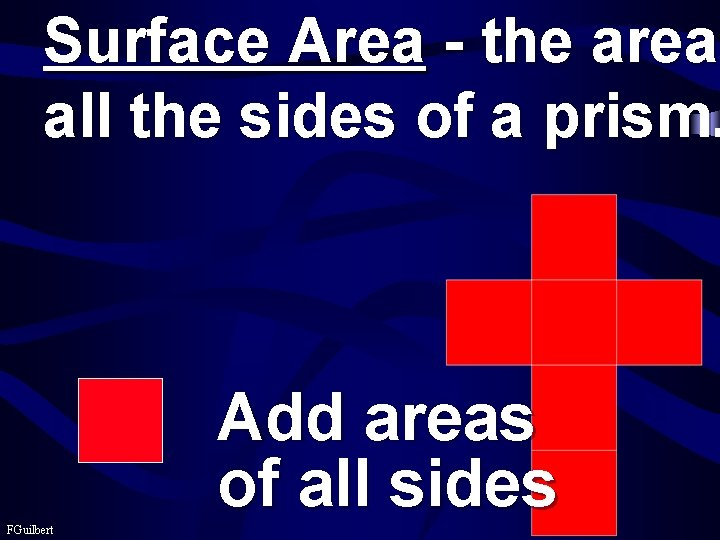Surface Area - the area all the sides of a prism. Add areas of all sides FGuilbert4 2 m 2 m 4 4 4 2 m 4 Add areas of all sides 4 2 FGuilbert 2 Area of each side = SA = 6 (4) = 2 24 mGeneral Formula SA= sum of area all sides side FGuilbert2 m 24 m 2 m 2 2 4 4 4 SA =2(2*2)+2(2*2 =2(2*2)+2(2* FGuilbert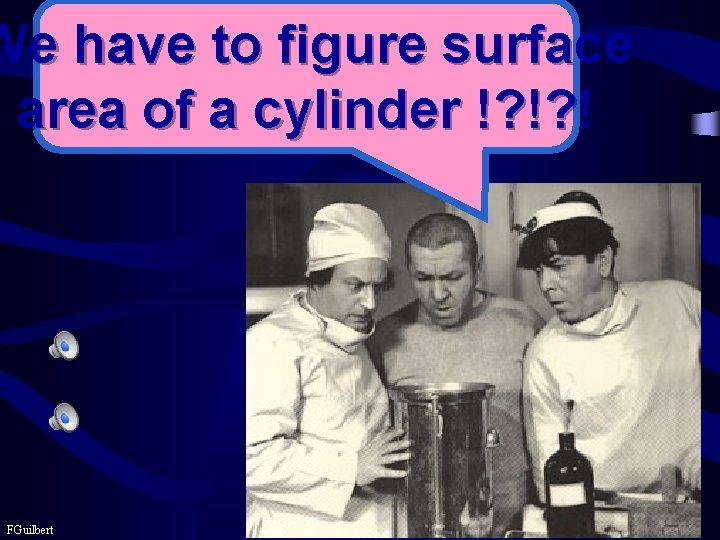We have to figure surface area of a cylinder !? !? ! FGuilbertFind S. A. of cylinder 10 m 9 m 10 m FGuilbert 9 m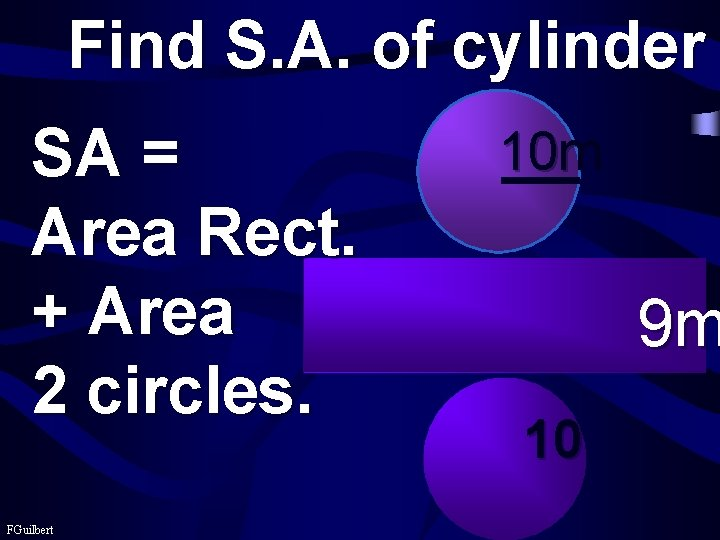Find S. A. of cylinder SA = Area Rect. + Area 2 circles. FGuilbert 10 m 9 m 10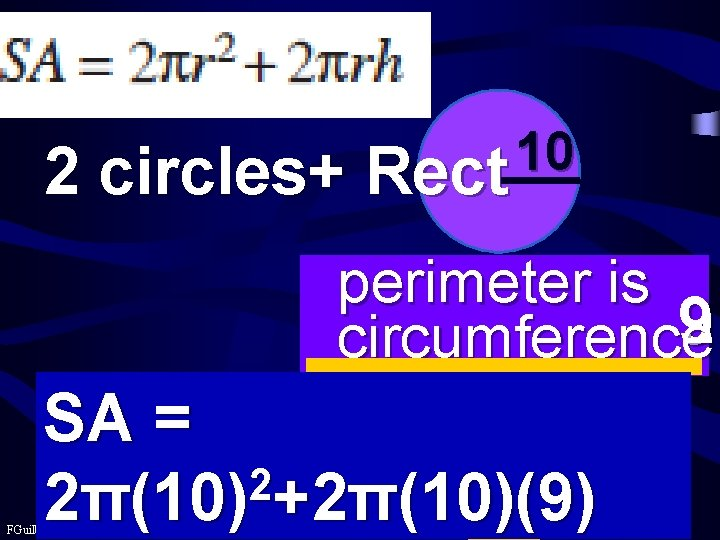10 2 circles+ Rect perimeter is 9 circumference SA = 10 2 2π(10) +2π(10)(9) FGuilbert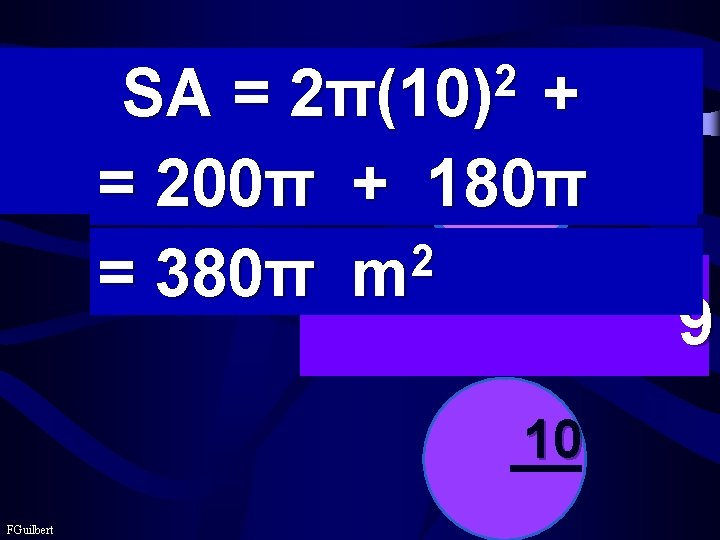2 2π(10) SA = + 10 2π(10)(9) = 200π + 180π 2 = 380π m 10 FGuilbert 9Seek first to understand, then to be understood. FGuilbert Stephen Covey